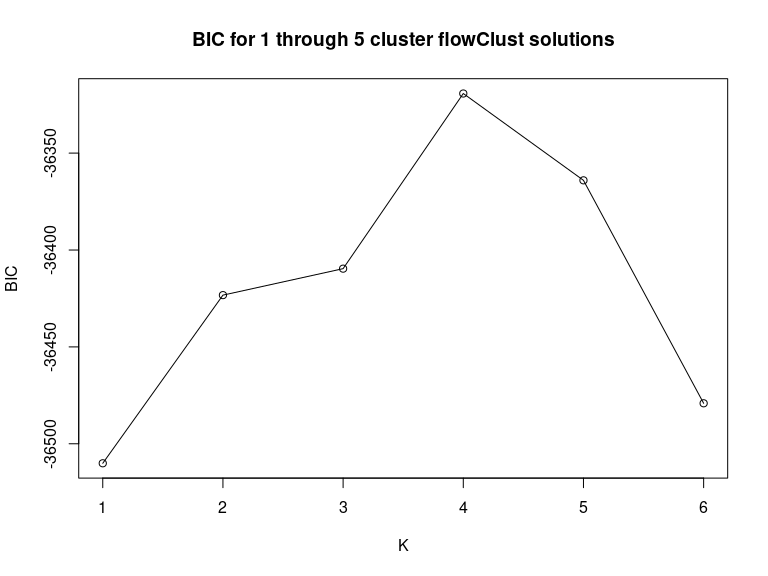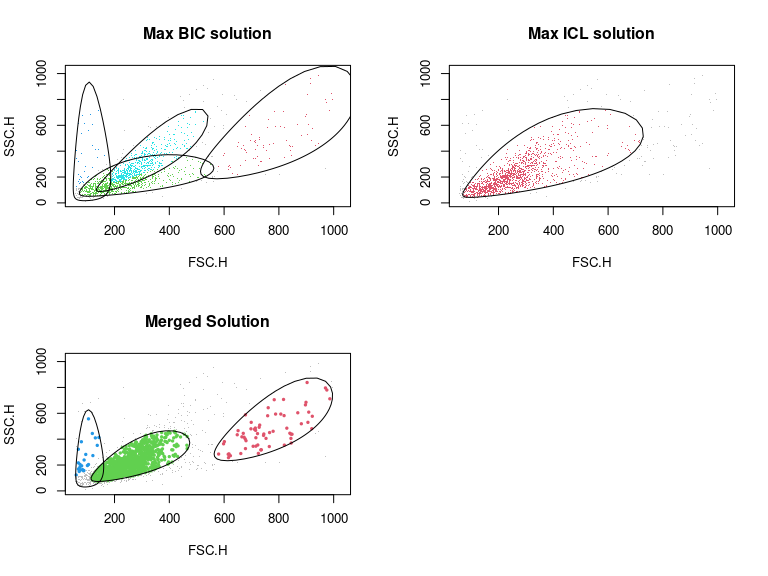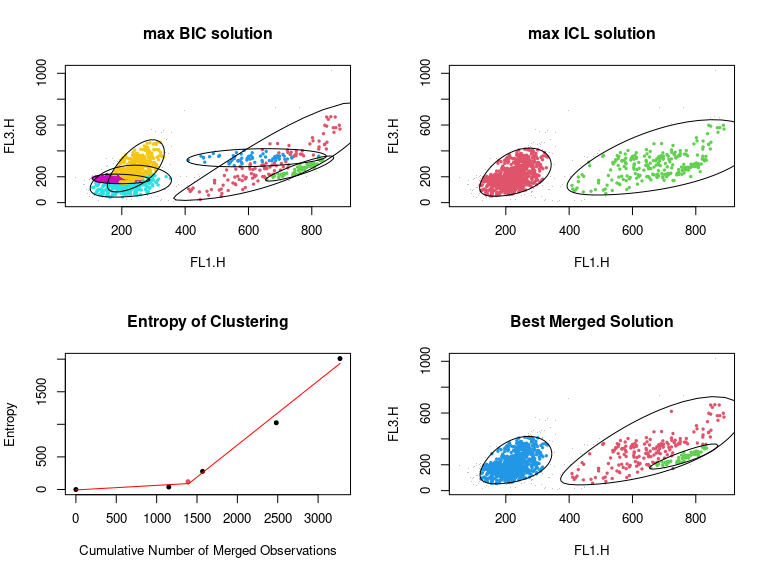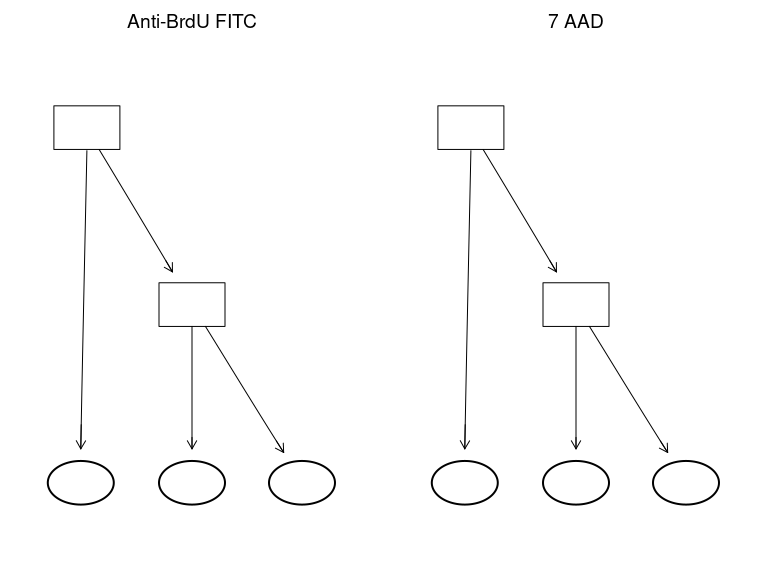# Merging Mixture Components for Cell Population Identification in Flow Cytometry Data The flowMerge package

## Licensing

Under the Artistic License, you are free to use and redistribute this software.

1. Greg Finak, Ali Bashashati, Ryan Brinkman, and Raphael Gottardo. Merging Mixture Components for Cell Population Identification in Flow Cytometry. Advances in Bioinformatics, vol. 2009, Article ID 247646, 12 pages, 2009. doi:10.1155/2009/247646

# Overview

We apply a cluster merging approach to perform automated gating of cell populations in flow cytometry data. The max BIC model fitting criterion for mixture models generally overestimates the number of cell populations (ie clusters) in flow cytometry data because the number of mixture components required to accurately model a distribution is usually greater than the number of distinct cell populations. Model fitting criteria based on the entropy, such as the ICL, provide better estimates of the number of clusters but tend to provide a poor fit to the underlying distribution. We combine these two approaches by merging mixture components from the max BIC fit based on an entropy criterion. This approach allows multiple mixture components to represent the same cell sub–population. Merged clusters are mixtures themselves and are summarized by a weighted combination of their component model parameters. The result is a mixture model that retains the good model fitting properties of the max BIC solution but the number of components more closely reflects the true number of distinct cell sub–populations.

# Installation

flowMerge requires several packages to be pre–installed for full functionality. Specifically, flowClust, flowCore, flowViz and snow (for parallel computations) should be installed and functional.

# Example: Cluster merging applied to the Rituximab data set

## The core function

To demonstrate the functionality we use a flow cytometry data set from a drug-screening project to identify agents that would enhance the anti-lymphoma activity of Rituximab, a therapeutic monoclonal antibody. The data set is an object of class flowFrame; it consists of eight variables, among them only the two scattering variables (FSC.H, SSC.H) and two channels for the fluorochrome measurements (FL1.H, FL3.H) are of interest in this experiment. The flowMerge package may be invoked upon the output of a call to flowClust, or it may be invoked on a flowFrame directly via the parallelized method pFlowMerge, which will itself call flowClust but throw away intermediate results. The following code will model one through 10 clusters using flowClust in a parallel manner using snow, choose the best BIC solution, merge clusters in the best BIC solution, choose the best merged solution based on the entropy criterion, then plot the results. This functionality is wrapped in the pFlowMerge function, with additional parallelization using snow. Additionally, if the snow cluster object passed to pFlowMerge is null, the non-parallel version of flowMerge is called.

library(flowMerge)
#>
#> Attaching package: 'BiocGenerics'
#> The following objects are masked from 'package:stats':
#>
#>     IQR, mad, sd, var, xtabs
#> The following objects are masked from 'package:base':
#>
#>     Filter, Find, Map, Position, Reduce, anyDuplicated, append,
#>     as.data.frame, basename, cbind, colnames, dirname, do.call,
#>     duplicated, eval, evalq, get, grep, grepl, intersect, is.unsorted,
#>     lapply, mapply, match, mget, order, paste, pmax, pmax.int, pmin,
#>     pmin.int, rank, rbind, rownames, sapply, setdiff, sort, table,
#>     tapply, union, unique, unsplit, which.max, which.min
#>
#> Attaching package: 'flowClust'
#> The following object is masked from 'package:BiocGenerics':
#>
#>     Map
#> The following object is masked from 'package:graphics':
#>
#>     box
#> The following object is masked from 'package:base':
#>
#>     Map
#>
#> Attaching package: 'flowMerge'
#> The following object is masked from 'package:snow':
#>
#>     checkForRemoteErrors
#> The following object is masked from 'package:stats':
#>
#>     BIC
data(rituximab)
summary(rituximab)
#>             FSC.H     SSC.H    FL1.H    FL2.H    FL3.H     FL1.A     FL1.W
#> Min.      59.0000   11.0000   0.0000   0.0000    1.000    0.0000   0.00000
#> 1st Qu.  178.0000  130.0000 197.0000  55.0000  150.000    0.0000   0.00000
#> Median   249.0000  199.0000 244.0000 116.0000  203.000    0.0000   0.00000
#> Mean     287.0822  251.8265 349.1638 126.3994  258.345   73.4589  17.59871
#> 3rd Qu.  331.0000  307.0000 445.0000 185.0000  315.000    8.0000   0.00000
#> Max.    1023.0000 1023.0000 974.0000 705.0000 1023.000 1023.0000 444.00000
#>             Time
#> Min.      2.0000
#> 1st Qu. 140.0000
#> Median  285.0000
#> Mean    294.0388
#> 3rd Qu. 451.0000
#> Max.    598.0000
flowClust.res <- flowClust(rituximab, varNames=c(colnames(rituximab)[1:2]), K=1:6,trans=1,nu.est=1,randomStart=20);

flowClust.res is a flowClust object containing the 5 cluster solution. We extract the BIC of each solution with the internal flowMerge function BIC:

plot(flowMerge:::BIC(flowClust.res),
main="BIC for 1 through 5 cluster flowClust solutions",xlab="K",ylab="BIC",type="o");flowClust.maxBIC<-flowClust.res[[which.max(BIC(flowClust.res))]];

Here we have extracted the max BIC solution, found for K=4. Before we run the cluster merging algorithm on the max BIC solution, we have to create a flowObj object from the flowClust result and the flowFrame data. We then run the merge function on the flowObj and extract then entropy of clustering from the merged results using the ENT function. The list of merged cluster solutions are input to the fitPiecewiseLinreg function. This function fits a piecewise linear regression to the entropy vs number of clusters and locates the position of the changepoint, if appropriate. Model selection is done via the BIC criterion. The results of the fit can be optionally plotted.

flowClust.flowobj<-flowObj(flowClust.maxBIC,rituximab);
flowClust.merge<-merge(flowClust.flowobj,metric="entropy");
#> Merged to 3 clusters
#> Merged to 2 clusters
#> Merged to 1 clusters
#> Updating model statistics
#> Rule of identifying outliers: 90% quantile
#> Updated model 1
#> Rule of identifying outliers: 90% quantile
#> Updated model 2
#> Rule of identifying outliers: 90% quantile
#> Updated model 3
#> Rule of identifying outliers: 90% quantile
#> Updated model 4
i<-fitPiecewiseLinreg(flowClust.merge);

Next we extract the merged solution with number of clusters equal to the position of the changepoint found by fitPiecewiseLinreg. This is the optimal merged solution based on the entropy criterion. The solution can be plotted with the plot method.

par(mfrow=c(2,2));
flowClust.mergeopt<-flowClust.merge[[i]];
plot(flowClust.res[],data=rituximab,main="Max BIC solution");
#> Rule of identifying outliers: 90% quantile
plot(flowClust.res[[which.max(flowMerge:::ICL(flowClust.res))]],data=rituximab,main="Max ICL solution");
#> Rule of identifying outliers: 90% quantile
plot(flowClust.mergeopt,level=0.75,pch=20,main="Merged Solution");
#> Rule of identifying outliers: 75% quantileWe see that the merged solution provides a better fit to the lymphocyte population than either the max BIC solution, or the max ICL solution. Additionally, debris and granulocytes are also clearly identified, in contrast to the ICL solution. We can extract the lymphocyte population and re-run flowClust and flowMerge on the fluorescence channels of the lymphocytes only.

pop<-which(apply(apply(getEstimates(flowClust.mergeopt)$locations,2,function(x)order(x,decreasing=TRUE)==2),1,all)); lymphocytes<-split(flowClust.mergeopt,population=list("lymphocytes"=pop))$lymphocytes;
lymphocytes<-lymphocytes[,c(3,5)];
l.flowC<-flowClust(lymphocytes,varNames=c("FL1.H","FL3.H"),K=1:8,B=1000,B.init=100,tol=1e-5,tol.init=1e-2,randomStart=50,nu=4,nu.est=1,trans=1);

The lymphocyte population is between the debris and the granulocytes in the forward and side–scatter dimensions. We can easily select it by examining the means of the three populations. We’re only interested in the fluorescence channels, so we subset those with the [] operator inherited from flowClust. Finally, another call to flowClust performs a round of clustering on the lymphocyte sub–population.

par(mfrow=c(2,2));
l.flowO<-flowObj(l.flowC[[which.max(flowMerge:::BIC(l.flowC))]],lymphocytes);
plot(l.flowO,main="max BIC solution",new.window=F,pch=20,level=0.9);
#> Rule of identifying outliers: 90% quantile
plot(flowObj(l.flowC[[which.max(flowMerge:::ICL(l.flowC))]],lymphocytes),main="max ICL solution",new.window=F,pch=20,level=0.9);
#> Rule of identifying outliers: 90% quantile
l.flowM<-merge(l.flowO);
#> Merged to 5 clusters
#> Merged to 4 clusters
#> Merged to 3 clusters
#> Merged to 2 clusters
#> Merged to 1 clusters
#> Updating model statistics
#> Rule of identifying outliers: 90% quantile
#> Updated model 1
#> Rule of identifying outliers: 90% quantile
#> Updated model 2
#> Rule of identifying outliers: 90% quantile
#> Updated model 3
#> Rule of identifying outliers: 90% quantile
#> Updated model 4
#> Rule of identifying outliers: 90% quantile
#> Updated model 5
#> Rule of identifying outliers: 90% quantile
#> Updated model 6
i<-fitPiecewiseLinreg(l.flowM,plot=T);
plot(l.flowM[[i]],new.window=F,main="Best Merged Solution",pch=20,level=0.9);#> Rule of identifying outliers: 90% quantile

We complete the analysis by choosing the best fitting merged solution and comparing it to the max BIC and max ICL solutions. ## Parallel computations with the snow package The methodology described in the first section is wrapped in a single function call with the additional benefits of utilizing the parallel processing capabilities of the snow package to speed up model fitting using flowClust. The function pFlowMerge can be passed multiple flowFrames in the form of a flowSet, or a list of flowFrames, as well as a snow cluster object, and the usual set of parameters required by flowClust to specify the model. The call parallelizes the computation of multiple models for each flowFrame. For example if one wants to fit all models starting from the one cluster model through to the ten cluster model for each of ten flowFrames, these will be distributed amongst the number of processors defined in the snow cluster object. Each processor will be assigned the calculation for a single flowFrame and model combination, until all processors are occupied. This speeds up computations significantly in a high–throughput analysis setting.

The resulting models are evaluated for the max BIC solutions for each flowFrame, the merging algorithm is run on each max BIC solution, the optimal merged solution is found as described in the example and returned to the user. The non–parallel version can be called via pFlowMerge with the cl’’ argument equal to NULL.

The flowMerge algorithm has been updated to increase speed and now supports parallelization when the foreach package is installed. FlowMerge now also supports merging on the mahalanobis distance metric as well as the entropy. These can be specified to the merging algorithm via the optional metric argument (metric=c("entropy","mahalanobis") to the merge() function. The default is to use “entropy”.

We have added some new features for plotting the tree of merged populations, and highlighting the nodes/populations according to marker expression. For the fluorescent markers above:

require(Rgraphviz)
f<-ptree("l.flowM",fitPiecewiseLinreg(l.flowM));
par(mfrow=c(1,2))
f(1);
#> A graphNEL graph with directed edges
#> Number of Nodes = 5
#> Number of Edges = 4
f(2);#> A graphNEL graph with directed edges
#> Number of Nodes = 5
#> Number of Edges = 4

Circular nodes with dashed borders show merged clusters. Elliptical nodes show the chosen populations in the best fitting model. Square nodes show the rest of the complete merging to a single cluster. Red indicated higher expression of the marker, and blue/green shows lower expression. Note that it is normalized between 0 and 1 on the range of the data.

## Upcoming improvements

FlowMerge is undergoing continuous usability improvements, specifically with respect to the parallel computation framework. Figures of merit and other statistics will be output to allow the user to monitor the success or failure of the merging algorithm as it works through a large data set. A framework for semi–supervised selection of lymphocyte populations across multiple samples is also in the works. pFlowMerge does not do any sophisticated memory management, as such if you have a large data set, we suggest feeding it to pFlowMerge piece by piece, depending on the amount of RAM available to your machine / cluster.

A: pFlowMerge is implemented to parallelize computations for flowSets or lists of flowFrames, rather than for multiple clusters in a single flowFrame. For example, if datais flowSet of ten flowFrames and cl is a snow cluster of ten nodes, then, running pFlowMerge(cl,flowSet,varNames=c("A","B","C"),K=1:10) will distribute the ten flowFrames across the ten nodes, and each node will evaluate the model for K=1:10 clusters. If you wish to parallelize the K=1:10 cluster computations across multiple nodes, you would need to make function call that utilizes snow functionality directly, such as: clusterMap(cl,function(...)try(flowClust(...)),list(flowFrame),varNames=list(c("A","B","C"),K=1:10). This will distribute the calculation of flowFrame for each value of K=1 through K=10 components across the ten nodes of cl. The addition of the try() wrapper ensures the function will return even if an individual model fails to converge for a given value of K. Alternately, if you wish to do the above for a flowSet you will need to replicate each element of the flowSet K$_{max$$} times, where K$$_{max$} is the total number of model components to evaluate perflowFrame. Again, an example:clusterMap(cl,function(…)try(flowClust(…)),rep(as(flowSet,“list”),each=length(Kvector)),varNames=list(c(“A”,“B”,“C”)),K=Kvector). Please remember to ensureflowClustis loaded in each R work environment on the nodes by callingclusterEvalQ(cl,library(flowClust)).